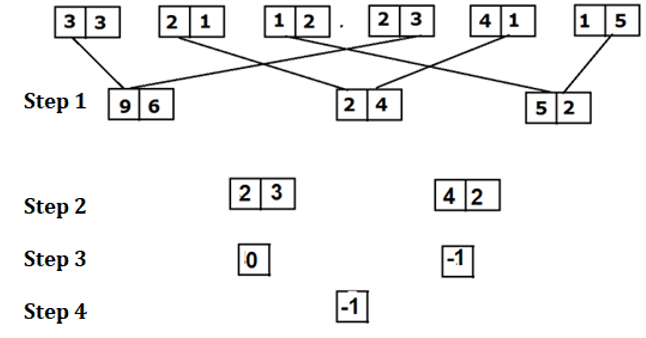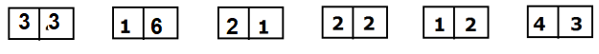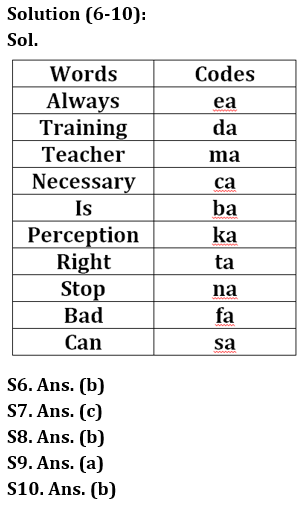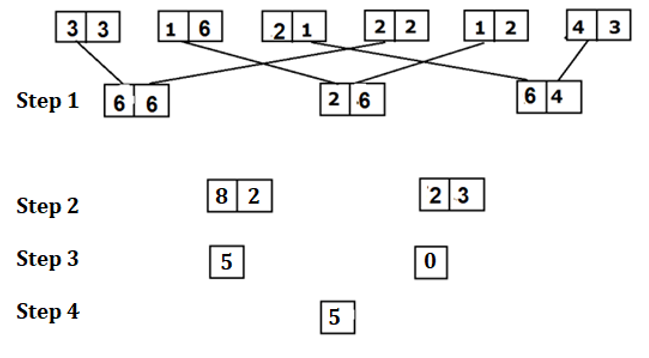Latest Banking jobs   »

# Reasoning Quiz For RBI Grade B Phase 1 2023-14th March

Direction (1-5): Study the following information carefully and answer the questions accordingly. The following words are coded in this manner:
‘Reasoning Mathematics English’ is coded as ‘I#7 N#19 V#8’
‘Word Letters Number’ is coded as ‘D@4 O#19 M@18’
‘Actors Singers Dancer’ is coded as ‘Z@19 H#19 W@18’
‘Obstacles Hurd Riddle’ is coded as ‘L#19 S@4 I@5’

Q1. What is the code for ‘Satellite’?
(a) H#5
(b) H@5
(c) E#5
(d) E@5
(e) None of these

Q2. ‘X@25’ is coded for which word?
(a) Crisis
(b) Cry
(c) Criteria
(d) Chronology
(e) None of these

Q3. What is the code for ‘Quality Analyst’?
(a) J@25 Z#20
(b) J#25 Z#20
(c) J#25 Z@20
(d) J@25 Z@20
(e) None of these

Q4. ‘G#25’ is coded for which word?
(a) Transistors
(b) Transactions
(c) Terminology
(d) Transport
(e) None of these

Q5. What is the code for ‘Verbal Ability’?
(a) E#12 Z@25
(b) E@12 Z#25
(c) E@25 Z#12
(d) E#25 Z#12
(e) None of these

Direction (6-10): Read the following information carefully to answer the questions given below.
In a certain code language,
‘Training is always necessary’ is written as ‘ba ca da ea’,
‘Teacher perception always right’ is written as ‘ea ka ma ta,
‘Teacher can stop training’ is written as ‘da ma na sa’
‘Stop bad perception necessary’ is written as ‘ka fa ca na’.

Q6. What is the code used for ‘right’?
(a) ea
(b) ta
(c) ka
(d) ma
(e) None of these

Q7. ‘fa’ is the code used for which of the following word?
(a) Stop
(b) Perception
(d) Necessary
(e) None of these

Q8. ‘ta ba fa’ are the code for which of the following words?
(a) Is right training
(d) Either (a) or (b)
(e) None of these

Q9. ‘Teacher is always linguist’ would be possibly written as ___?
(a) ba ma pa ea
(b) da ca ea ma
(c) ea ba ma na
(d) ba na ka ca
(e) None of these

Q10. Which word is represented by ‘na’?
(a) Necessary
(b) Stop
(c) Right
(d) Perception
(e) None of these

Directions (11-15): An input-output is given in different steps. Some mathematical operations are done in each step. No mathematical operation is repeated in next step.As per the rules followed in the steps given above, find out in each of the following questions the appropriate step for the given input.Q11. Which of the following combinations will come in step III of the given input?
(a) 3, -2
(b) -2, 4
(c) 2, -1
(d) 5, 0
(e) 2, 8

Q12. Find the addition of numbers obtained in Step II?
(a) 45
(b) 48
(c) 105
(d) 57
(e) 65

Q13. Find the difference between the two numbers obtained in Step III?
(a) 3
(b) 4
(c) -3
(d) 2
(e) 5

Q14. If the last step number is multiplied by 4 what will be the final answer?
(a) 15
(b) 20
(c) -16
(d) 12
(e) None of the above

Q15. If all the digits of each block in step I are added, then what will be the answer?
(a) 26
(b) 30
(c) 28
(d) 32
(e) None of the above

Solutions

Solution (1-5):
Sol. For Letters:
Reverse letter of the first letter of each word according to English alphabet. Ex- Reverse of A is Z.
For Symbols: @ for even number of letters in the word and # for odd number of letters in the word.
For Numbers: Place value of the last letter of each word according to English alphabet. Ex- place value of A is 1.

S1. Ans. (a)
S2. Ans. (d)
S3. Ans. (b)
S4. Ans. (c)
S5. Ans. (b)Solution (11-15):
Step-1
1. First digit of the first block has to be multiplied with the second digit of the fourth block.
2. Second digit of the first block has to be multiplied with the first digit of fourth block.
Step 2:
1. First digit of all numbers has to added in step I and then multiply by 2 and write down the value in reverse order.
2. Add the second digit of all numbers in step I and then multiply by 2 and write down the value in reverse order.
Step 3:
1. Second digit of second block has to be subtracted from the first digit of the first block.
2. Second digit of first block has to be subtracted from the Second digit of the first block.
Step 4: Addition of both the numbers.S11. Ans.(d)
S12. Ans.(c)
S13. Ans.(e)
S14. Ans.(b)
S15. Ans.(b)## FAQs

### What is the selection process of the Bank Clerk?

There are 4 sections in the RBI Grade B Phase 1 Exam i.e. English Language, General Awareness, Quantitative Aptitude & Reasoning.

#### Congratulations!Union Budget 2023-24: Free PDF